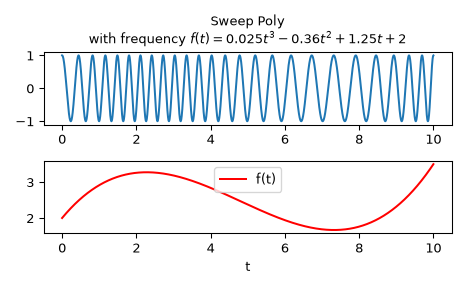# scipy.signal.sweep_poly¶

scipy.signal.sweep_poly(t, poly, phi=0)[source]

Frequency-swept cosine generator, with a time-dependent frequency.

This function generates a sinusoidal function whose instantaneous frequency varies with time. The frequency at time t is given by the polynomial poly.

Parameters
tndarray

Times at which to evaluate the waveform.

poly1-D array_like or instance of numpy.poly1d

The desired frequency expressed as a polynomial. If poly is a list or ndarray of length n, then the elements of poly are the coefficients of the polynomial, and the instantaneous frequency is

`f(t) = poly*t**(n-1) + poly*t**(n-2) + ... + poly[n-1]`

If poly is an instance of numpy.poly1d, then the instantaneous frequency is

`f(t) = poly(t)`

phifloat, optional

Phase offset, in degrees, Default: 0.

Returns
sweep_polyndarray

A numpy array containing the signal evaluated at t with the requested time-varying frequency. More precisely, the function returns `cos(phase + (pi/180)*phi)`, where phase is the integral (from 0 to t) of `2 * pi * f(t)`; `f(t)` is defined above.

Notes

New in version 0.8.0.

If poly is a list or ndarray of length n, then the elements of poly are the coefficients of the polynomial, and the instantaneous frequency is:

`f(t) = poly*t**(n-1) + poly*t**(n-2) + ... + poly[n-1]`

If poly is an instance of `numpy.poly1d`, then the instantaneous frequency is:

`f(t) = poly(t)`

Finally, the output s is:

`cos(phase + (pi/180)*phi)`

where phase is the integral from 0 to t of `2 * pi * f(t)`, `f(t)` as defined above.

Examples

Compute the waveform with instantaneous frequency:

```f(t) = 0.025*t**3 - 0.36*t**2 + 1.25*t + 2
```

over the interval 0 <= t <= 10.

```>>> from scipy.signal import sweep_poly
>>> p = np.poly1d([0.025, -0.36, 1.25, 2.0])
>>> t = np.linspace(0, 10, 5001)
>>> w = sweep_poly(t, p)
```

Plot it:

```>>> import matplotlib.pyplot as plt
>>> plt.subplot(2, 1, 1)
>>> plt.plot(t, w)
>>> plt.title("Sweep Poly\nwith frequency " +
...           "\$f(t) = 0.025t^3 - 0.36t^2 + 1.25t + 2\$")
>>> plt.subplot(2, 1, 2)
>>> plt.plot(t, p(t), 'r', label='f(t)')
>>> plt.legend()
>>> plt.xlabel('t')
>>> plt.tight_layout()
>>> plt.show()
```